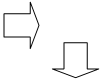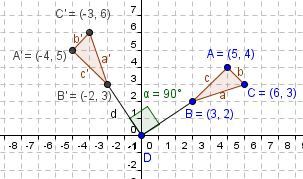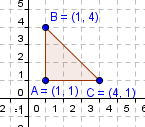Email us to get an instant 20% discount on highly effective K-12 Math & English kwizNET Programs!

#### Online Quiz (WorksheetABCD)

Questions Per Quiz = 2 4 6 8 10

### Geometry7.5 Rotation

 Rotation turns a figure about a fixed point, called the center of rotation. Rays drawn from the center of rotation to a point and its image form the angle of rotation. Rotations can be clockwise or counterclockwise.Coordinate Rules for Rotations about the origin: When a point (a,b) is rotated counterclockwise about the origin, the following rules are true: For a rotation of 900 (x,y) � (-y, x). For a rotation of half turn or 1800 (x,y) � (-x, -y). For a rotation of 2700 (x,y) � (y, -x). When a point (a,b) is rotated clockwise about the origin, the following rules are true: For a rotation of 900(x,y) � (y, -x). For a rotation of half turn or 1800 (x,y) � (-x, y). Examples of figures rotated counterclockwise direction 900, 1800 and 2700:Directions: Use a graph paper and rotate the following figures for 900, 1800 and 2700 about the origin. Also list the coordinates of the vertices of the image:Using above data and choose the correct answer. Also write at least 5 examples of your own.
 Q 1: Find the image of (3,4) in the 900 clockwise direction.(3,-4)(4,-3)(-4,3) Q 2: Find the image of (3, 5) in the 900 counterclockwise direction.(-5,3)(3,-5)(-3,5) Q 3: Find the image of (5,8) in the half turn about the origin.(-5,-8)(-5,8)(5,-8) Q 4: Find the image of (5,6) in the 900 clockwise direction.(6,-5)(-6,5)(-5,6) Q 5: Find the image of (4,5) in the 900 clockwise direction.(5,-4)(-4,5)(-5,4) Q 6: Find the image of (3,4) in the half turn about the origin.(-3,4)(3,-4)(-3,-4) Question 7: This question is available to subscribers only! Question 8: This question is available to subscribers only!